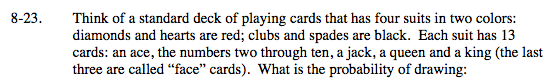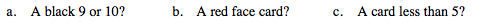### Home > MC2 > Chapter 8 > Lesson 8.1.2 > Problem8-23

8-23.$\frac{4}{52}$

There are 2 black suits for each card.
So there are 2 black 9's and 2 black 10's.

Can you reduce this number?

There are 2 red suits, and each suit has 3 face cards.

$\frac{\text{number of red face cards}}{\text{number of total cards in a deck}}$

$\frac{4}{13}$

There are 4 suits. Within each suit,how many cards are less than 5?